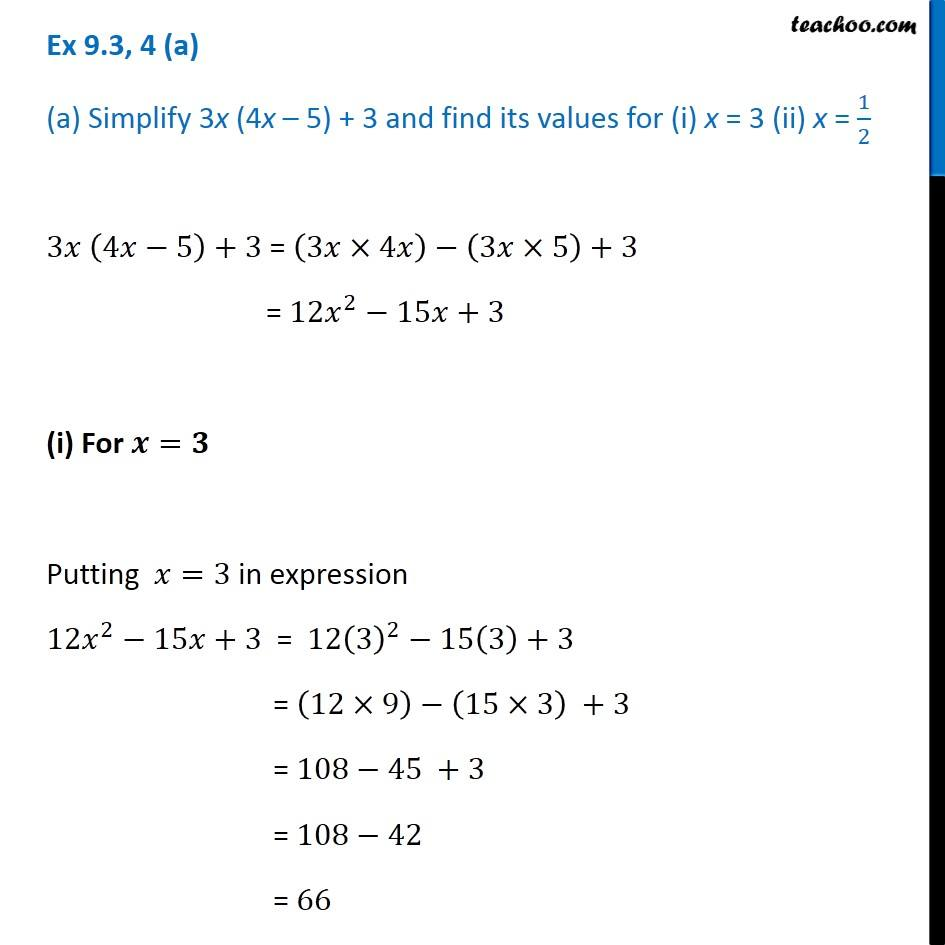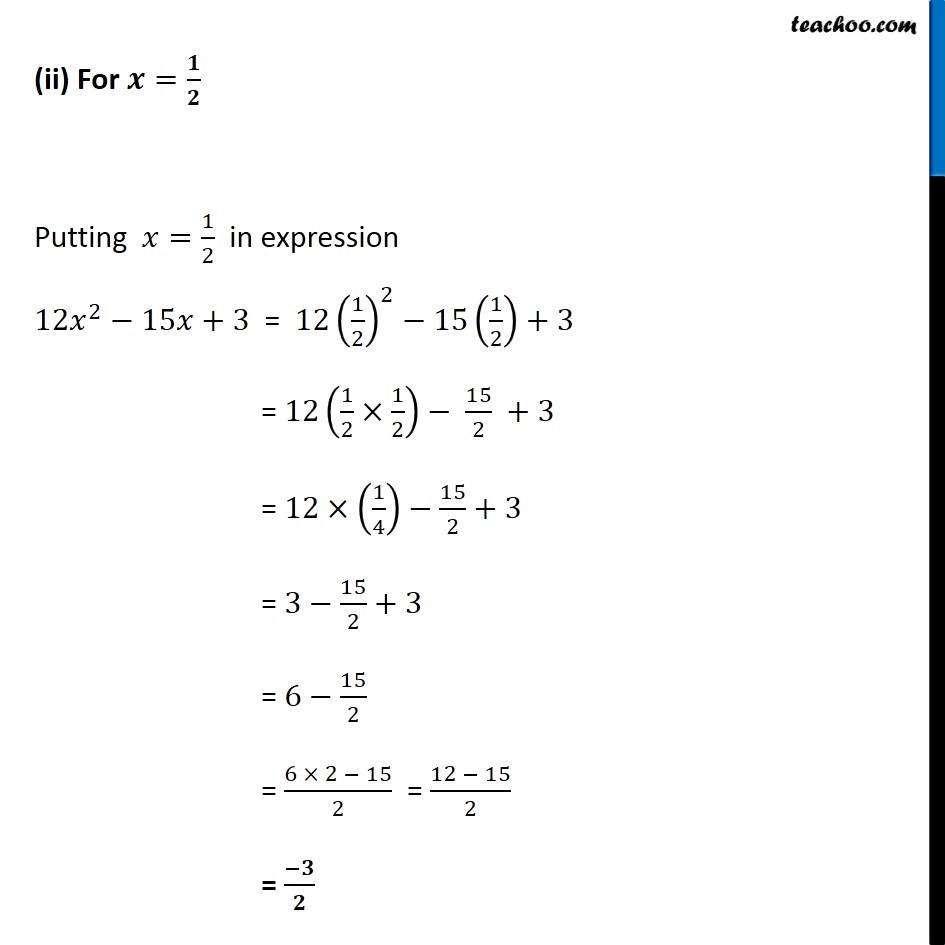Multiplication of Monomials by Binomials and Trinomials

Chapter 8 Class 8 Algebraic Expressions and Identities
Concept wiseLearn in your speed, with individual attention - Teachoo Maths 1-on-1 Class

### Transcript

Ex 8.3, 4 (a) (a) Simplify 3x (4x – 5) + 3 and find its values for (i) x = 3 (ii) x = 1/2 3𝑥 (4𝑥−5)+3 = (3𝑥×4𝑥)−(3𝑥×5)+3 = 12𝑥^2−15𝑥+3 (i) For 𝒙=𝟑 Putting 𝑥=3 in expression 12𝑥^2−15𝑥+3 = 12(3)^2−15(3)+3 = (12×9)−(15×3) +3 = 108−45 +3 = 108−42 = 66 (ii) For 𝒙=𝟏/𝟐 Putting 𝑥=1/2 in expression 12𝑥^2−15𝑥+3 = 12(1/2)^2−15(1/2)+3 = 12(1/2×1/2)− 15/2 +3 = 12×(1/4)−15/2+3 = 3−15/2+3 = 6−15/2 = (6 × 2 − 15)/2 = (12 − 15)/2 = (−𝟑)/𝟐